# Fast Order Entry to Split the Spread

Find unique algos, price and size tools to help you easily and quickly submit many types of orders, including "split spread" orders. Split spread refers to any order priced within the spread between the bid and ask price. When they fill, these orders yield significant price improvement, and because they typically add liquidity, split spread orders may also earn exchange rebates.

Quickly and easily submit split spread orders from the desktop Order Entry Panel.

## Desktop - Order Entry Panel

Our innovative order entry tools, including the Adaptive Algo, the Price Line, Relative Price Buttons and the Price Wand, let you interact quickly with prices and sizes, setting new standards in speed and precision for placing orders within the spread.Convert the Adaptive Market into an Adaptive Limit order by setting a limit price quickly and precisely with our revolutionary Order Entry price tools. An adaptive limit order goes in near the bid price (for a buy) or the ask price (for a sell), and waits to fill. If it doesn't fill, it is resubmitted at a slightly more aggressive price. It continues this pattern of "wait and move" within the spread until it fills, is canceled, or hits the limit price cap/floor.

#### Price Line

The Price Line lets you set a limit price at the midpoint of the bid/ask spread with a single click.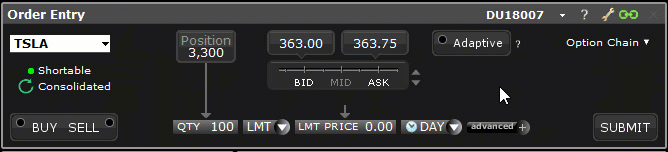#### Relative Price Buttons

Click the up/down buttons next to the Price Line to display Relative Price Buttons. These allow you to set the order price relative to the bid or ask at the time of the order. If you select the "% of Spread" or "+/- Tick" price mode, it will become the primary price line mode until you make a new selection.

When hovering over the relative price, the tool will show you the calculated price which would be used when clicking.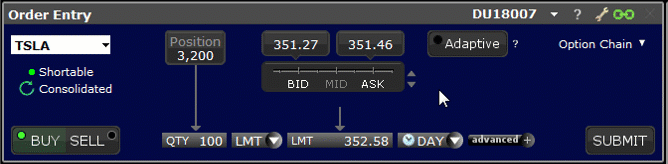Choose a percent of the bid/ask spread to have the system calculate an order price to capture a portion of the spread. The available percentages are calculated at the time the tool is loaded.

#### +/- Tick

Choose a tick value that is added to or subtracted from the bid or ask price at the time you create the order.

#### Relative Price Wand

Click the price field and use the price wand to select a price. The wand comprises two sections: absolute prices and relative prices. The Price Wand is available throughout the platform for instruments with real time market data anywhere you can enter an order.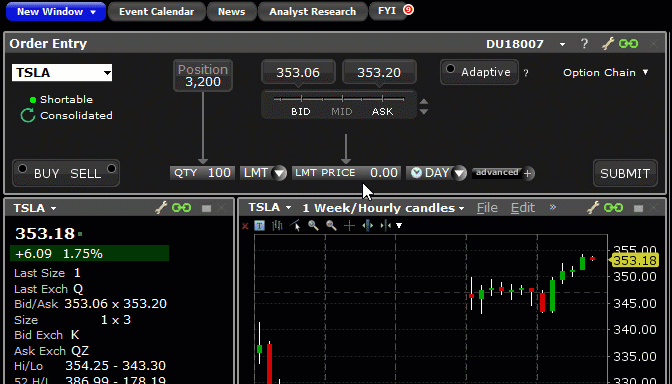Increments in the wand are shaded to provide a quick visual queue. Values at the bid and below are shaded light blue, and values at the ask and above are shaded light red. Remove shading by deselecting the feature "Color entries in Price Wand based on bid/ask" from the Orders > Settings page of Global Configuration.

###### Absolute Prices

Select a price from the left column of the Price Wand to set a limit price.

###### Relative Prices

The right side of the Price Wand displays relative prices. Click the toggle button at the bottom of the prices to switch views between "% of Spread" and "+/- Tick." Click a relative price to have the system calculate the absolute order price.

Increments that are displayed for both "% of Spread" and "+/- Tick" values in the Relative Price buttons and the Price Wand are determined based on the width of the spread at the time the tool is opened, which could be when you choose a relative price tool, or when you load an instrument if your Order Entry panel is already in Relative Price mode.

When you select a relative price, the system creates a one-time order price that is based on the spread at the time the tool is loaded and the bid or ask price at the time you create the order.

See " Understanding Relative Prices" below for details on how relative price increments and relative order prices are determined.

## Understanding Relative Prices

The Relative Price tools offer a novel way to define a limit price by offering values that are relative to the bid or ask price. Relative prices are then used to determine a corresponding absolute order price. The absolute order price derived from the relative price is calculated one time, using the current bid or ask at the time you create the order. It is not updated if prices change.

Relative prices are displayed in a series of increments determined by the instrument's current spread.

• For values BELOW the midpoint of the bid/ask, prices are relative to the bid price.
• For values ABOVE the midpoint, prices are relative to the ask.

There are two basic components to the relative pricing model: the price increment ladder, and the relative price.

Price increments are calculated based on the bid/ask spread at the time a relative price tool is loaded. This means that the increments will vary between different instruments, and also for the same instrument at different times if the width of the spread has changed. You can refresh increments by clicking or tapping the relative icon to reload the tool.

The increment ladder can be viewed as tick increments, or as a "% of the spread." Toggle between these views using the "%" and coin icons at the bottom of the relative price display.

###### Tick Increments

For the "+/- Ticks" ladder, we generally display all ticks to ensure a sufficient range of prices is offered. In some cases and for products with wider spreads, ticks will be hidden based on the volatility of the instrument to ensure efficiency.

The % of spread Relative Price Increments are calculated using the instrument's minimum tick size and the width of the bid/ask spread at the time you load the tool.

`Relative Price Increment (in %) = Minimum tick size / spread x 100%`

If the % of spread increment calculation results in a ratio less than 10%, we will use 10%.

###### Examples

Let's take a look at a few examples showing how the "% of spread" price ladder would populate based on the width of the bid/ask spread.

Examples all assume a minimum tick size of 1 penny.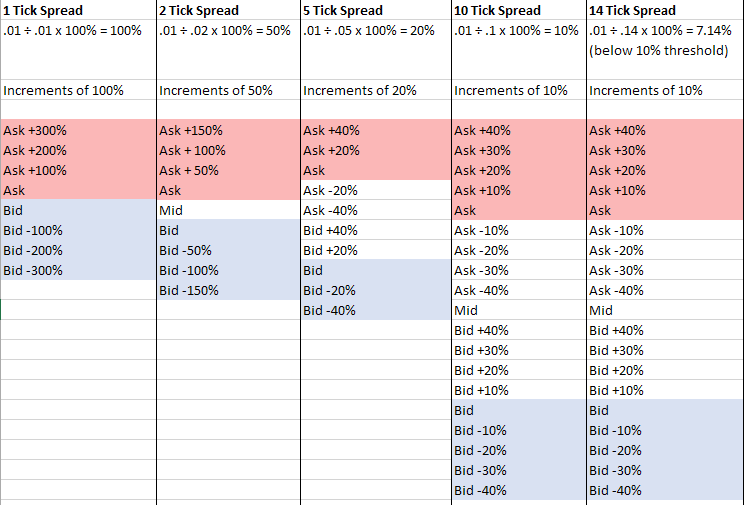In other words, when the bid/ask spread is 1 tick wide, that 1 tick is 100% of the spread. If the spread is 5 ticks wide, each tick is 20% of the spread. Wider spreads offer more price increments between the bid and ask prices. In cases where the spread is wide, the increments for absolute prices will not be the same as the increments for relative prices (since we round once the spread ratio is less than 10%).

#### Relative Prices

When you select a relative price, we calculate the absolute order price using the current bid/ask price, and the bid/ask spread at the time the tool was loaded. This means that the width of the spread used to determine price increment and the width of the spread at the time the price is applied could be different.

The calculation for determining the absolute price from the relative price is:

`Price +/- (loaded spread (as a percent) x relative increment (as a percent))`

In the example illustrated below, the spread was 3 ticks wide when the % of spread wheel was loaded, resulting in increments of 33%.

If I select "Ask -33%" and the ask price is 171.92, the absolute price would be:

`171.92 – (.03 x .33) = 171.91`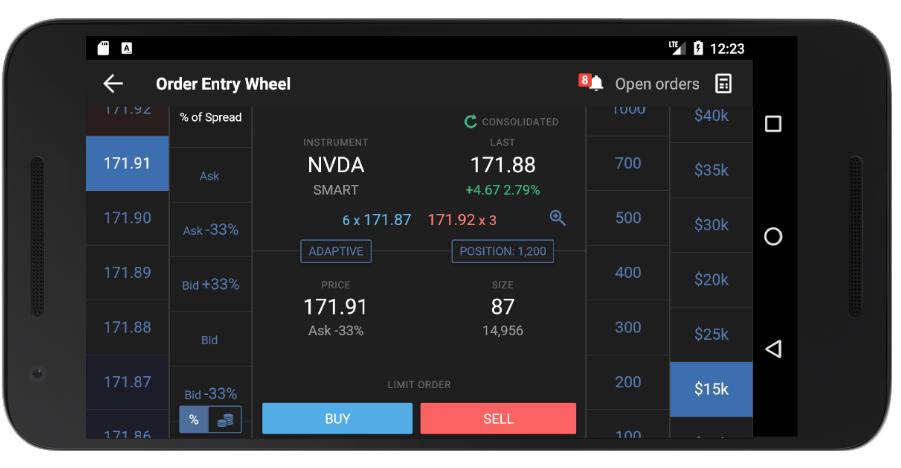Note that the corresponding absolute price is highlighted in the absolute price wheel and populates the Price field.

When you select a price and size value in the Mobile Order Wheel, the absolute value for each selection populates the Price and Size fields and the corresponding relative values are shown below the absolute values. The relative price value changes to reflect the current relationship of the absolute order price to changes in the bid or ask.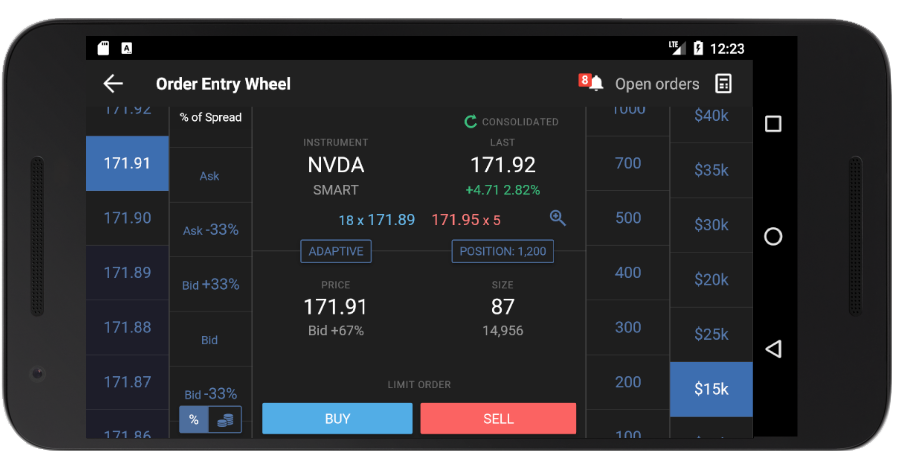Continuing the example we started above - the absolute price of 171.91 was calculated using the "Ask -33%" relative price when the ask price was 171.92. When the ask price changes to 171.95 as shown above, your order price remains the same at 171.91, it now has a new relationship with the bid/ask which is shown in the relative price below the order price. In this case, the price of 171.91 is now below the new bid/ask midpoint, and so is considered relative to the bid price; specifically, "Bid +67%."

The risk of loss in online trading of stocks, options, futures, currencies, foreign equities, and fixed income can be substantial. Options are not suitable for all investors. For more information, read the "Characteristics and Risks of Standardized Options".